﻿ 基于数据深度挖掘的船体吃水度监测系统设计与实现
 舰船科学技术2022, Vol. 44Issue (8): 186-189    DOI: 10.3404/j.issn.1672-7649.2022.08.040PDF

Design and implementation of hull draft monitoring system based on data depth mining
LI Shu-yi
School of College of Life and Health Science, Northeastern University, Shenyang 110000, China
Abstract: When the ship is sailing in the inland waterway, when the hull draft is too large, the collision and grounding accidents between the ship and the waterway are easy to occur, resulting in the loss of life and property. Therefore, it is necessary to monitor the ship draft state in the Inland Waterway in real time. In this paper, an online hull draft monitoring system is designed. The system uses ultrasonic sensor to obtain the hull draft data, and combines data mining technology to classify and identify the massive ship draft data, so as to improve the accuracy of hull draft data and ensure the safety of inland shipping traffic.
Key words: data mining     the depth of the draft     online monitoring
0 引　言

1 船体吃水度监测系统的设计 1.1 总体框架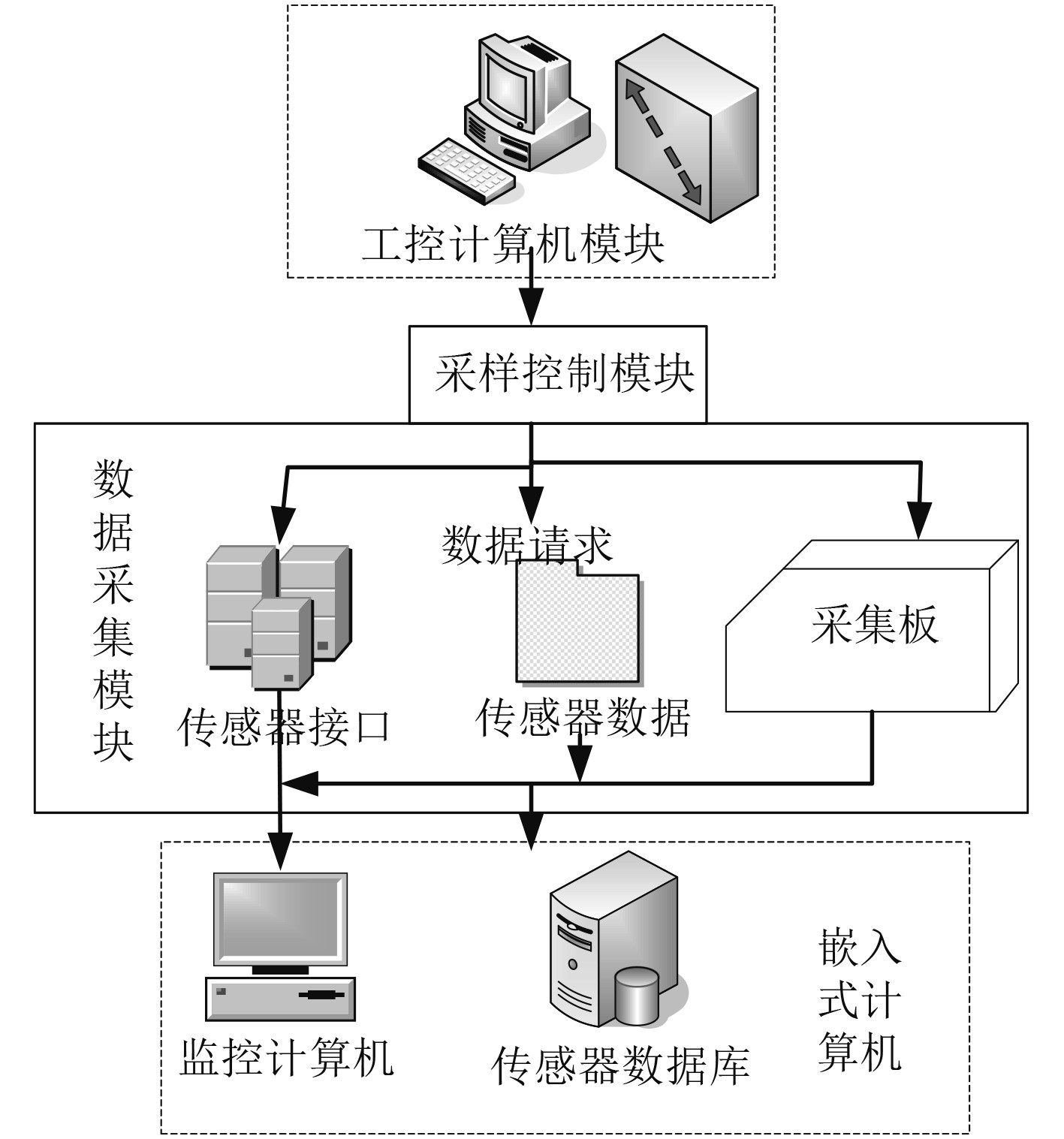图 1 内河航道的船体吃水深度监测系统原理图 Fig. 1 Schematic diagram of hull draft monitoring system in inland waterway

1）工控计算机模块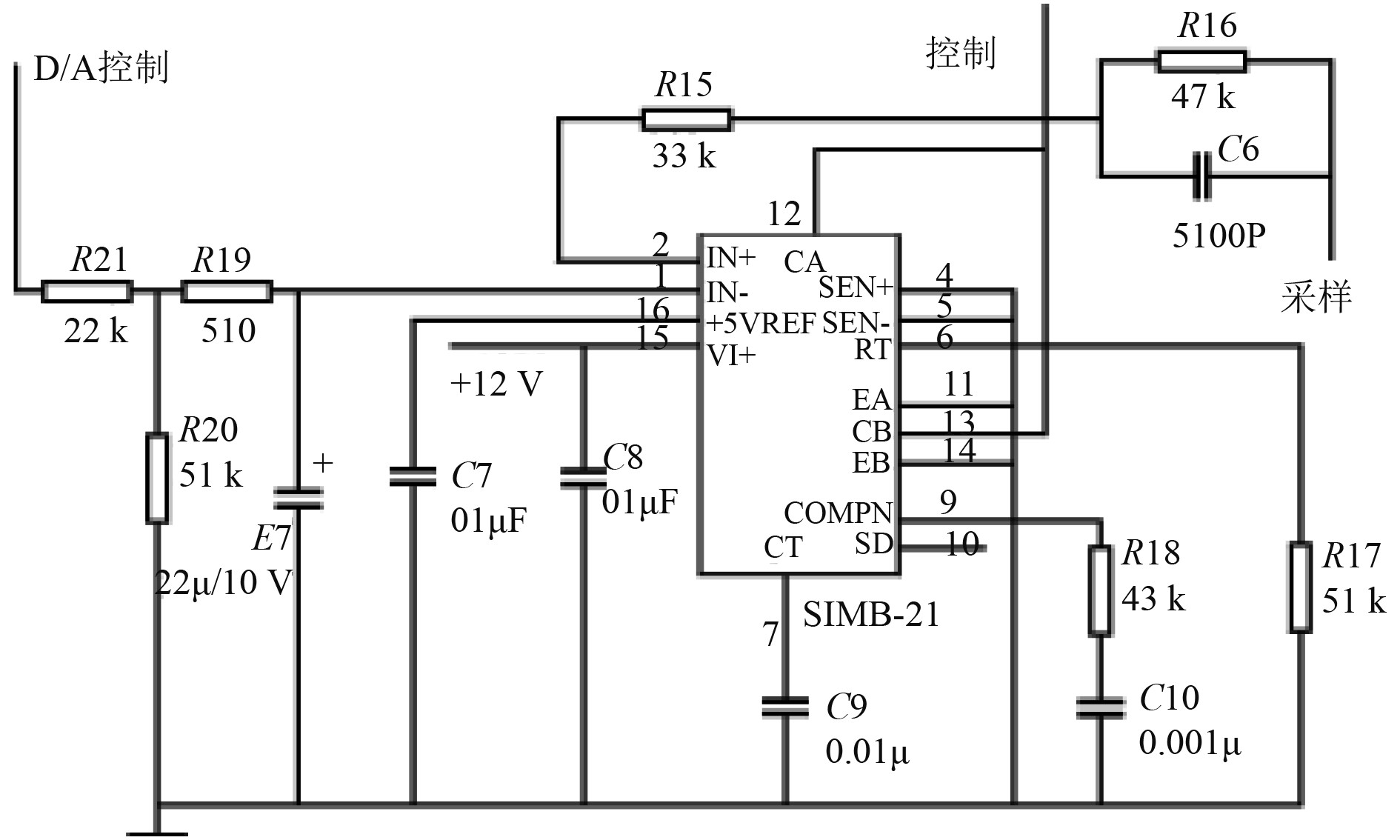图 2 船体吃水深度监测系统的工控机连接电路 Fig. 2 Industrial computer connection circuit of hull draft monitoring system

①参数设置功能

②数据库管理功能

③数据交换功能

④显示与报警功能

2）采样控制模块

3）数据采集模块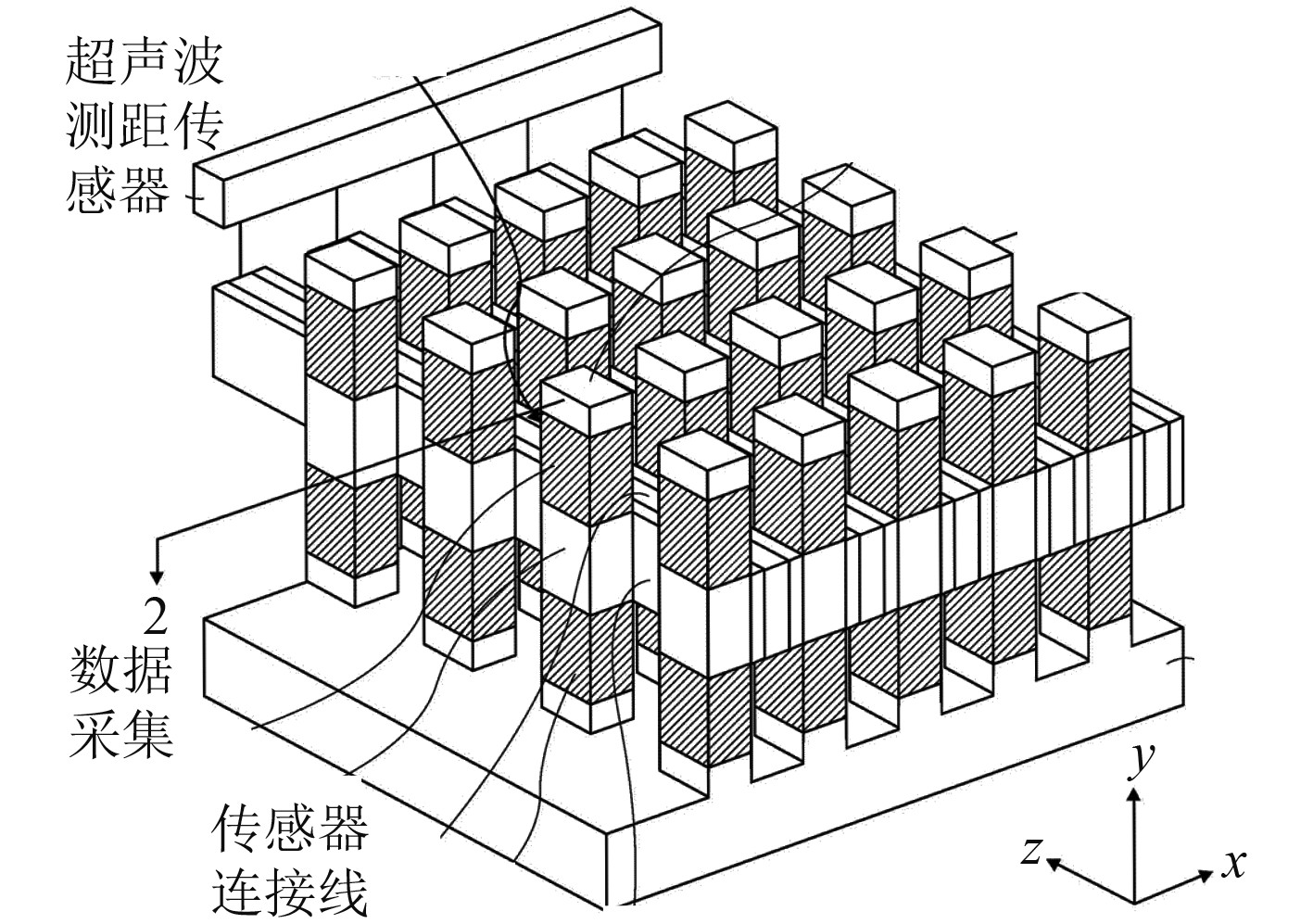图 3 水下船体吃水监测超声波传感器阵列示意图 Fig. 3 Schematic diagram of ultrasonic sensor array for underwater hull draft monitoring

 $c = 1449.2 + 4.6T + \frac{{(1.34 - 0.01T)}}{{(S - 35)}} + 0.06D \text{。}$

 $p = \frac{1}{{{R_1}}}{e^{ - wt}} \text{。}$

 $\begin{array}{*{20}{c}} {{\nabla ^2}p + {k^2}p = 0} ，\\ {{\nabla ^2}p - \dfrac{1}{{{c^2}}}\dfrac{{{\partial ^2}p}}{{\partial {t^2}}} = - 4{\text{π}} \delta (t){e^{jwt}}} \text{。} \end{array}$

 ${D_i} = \sqrt {{{\left( {{x_t} - x} \right)}^2} + {{\left( {{y_i} - y} \right)}^2} + {{\left( {{z_i} - z} \right)}^2}} \text{。}$

 ${t_i} = \frac{{{D_i}}}{c} 。$

4）嵌入式计算机模块

1.2 船舶吃水监测系统的信号采集电路设计

1）数据滤波功能

FPGA芯片外接中值滤波电路，可对超声波传感器采集的吃水深度数据进行中值滤波，将数据中的噪声、干扰信号剔除，从而提高船体吃水深度数据的采集精度。

2）ARM通信功能

FPGA芯片具备ARM通信功能，将系统采集的船体吃水数据发送至系统的工控机

3）信号转换功能。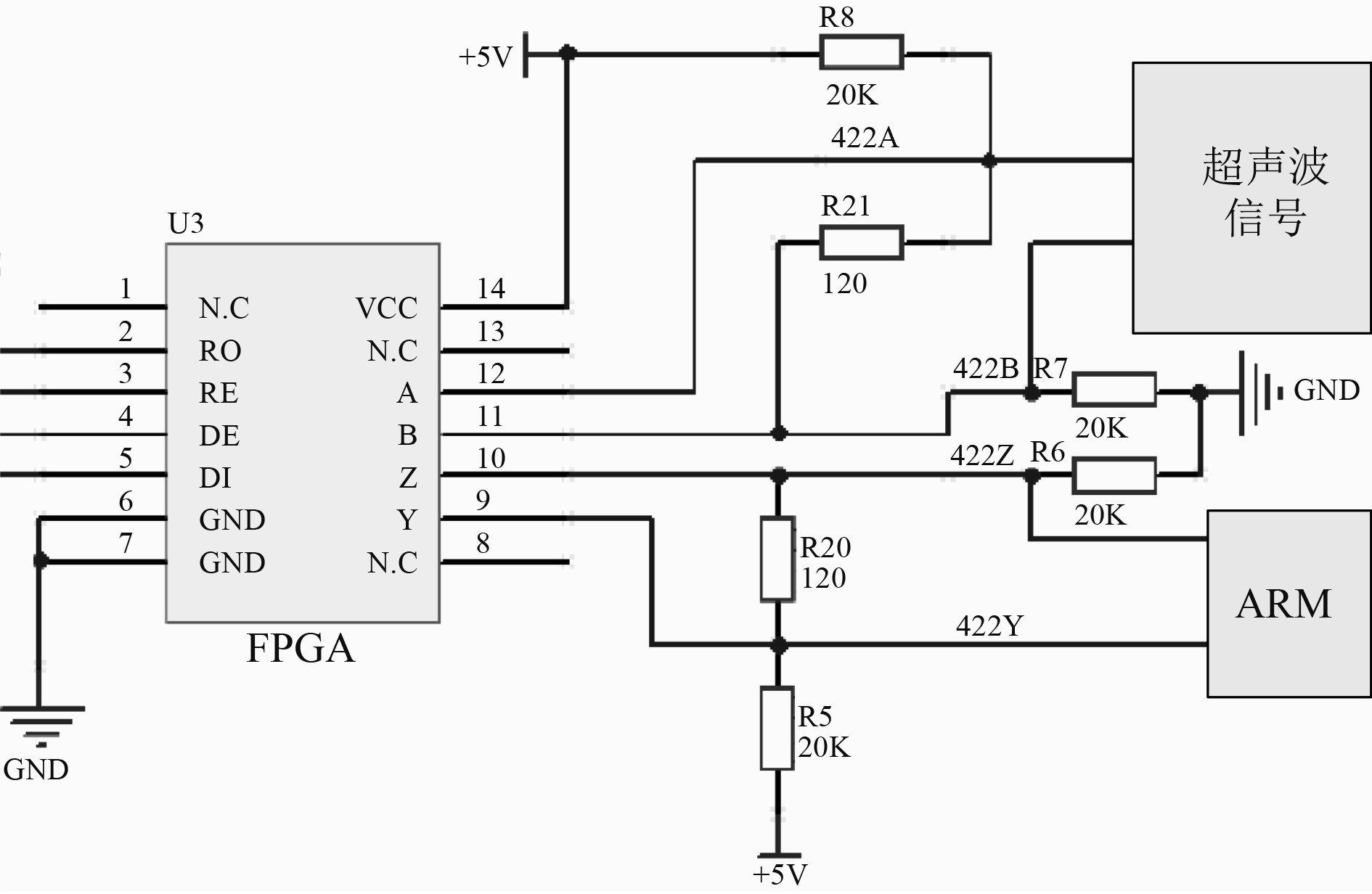图 4 吃水监测系统的FPGA芯片电路图 Fig. 4 FPGA chip circuit diagram of draft monitoring system

 ${f_i} = {med} \left( {{y_{i - N}},{y_{i - (N - 1)}}, \cdots ,{y_{i + N - 1}},{y_{i + N}}} \right) \text{，}$

 ${W_N} = \{ - N,, \cdots ,0, \cdots ,N - 1,N\} \text{，}$

 ${\hat \sigma _{out}} = \frac{1}{{4L{f^2}(0)}},L > 1。$

2 基于数据深度挖掘的船体吃水监测技术开发 2.1 基于最佳阈值理论的吃水深度数据挖掘

1）监测系统采集数据的初始化

 $g = {{\rm{int}}} \left( {\frac{{{h_{\max }} - {h_{\min }}}}{{dh}}} \right) \text{。}$

2）获取船舶吃水深度离散数据的统计直方图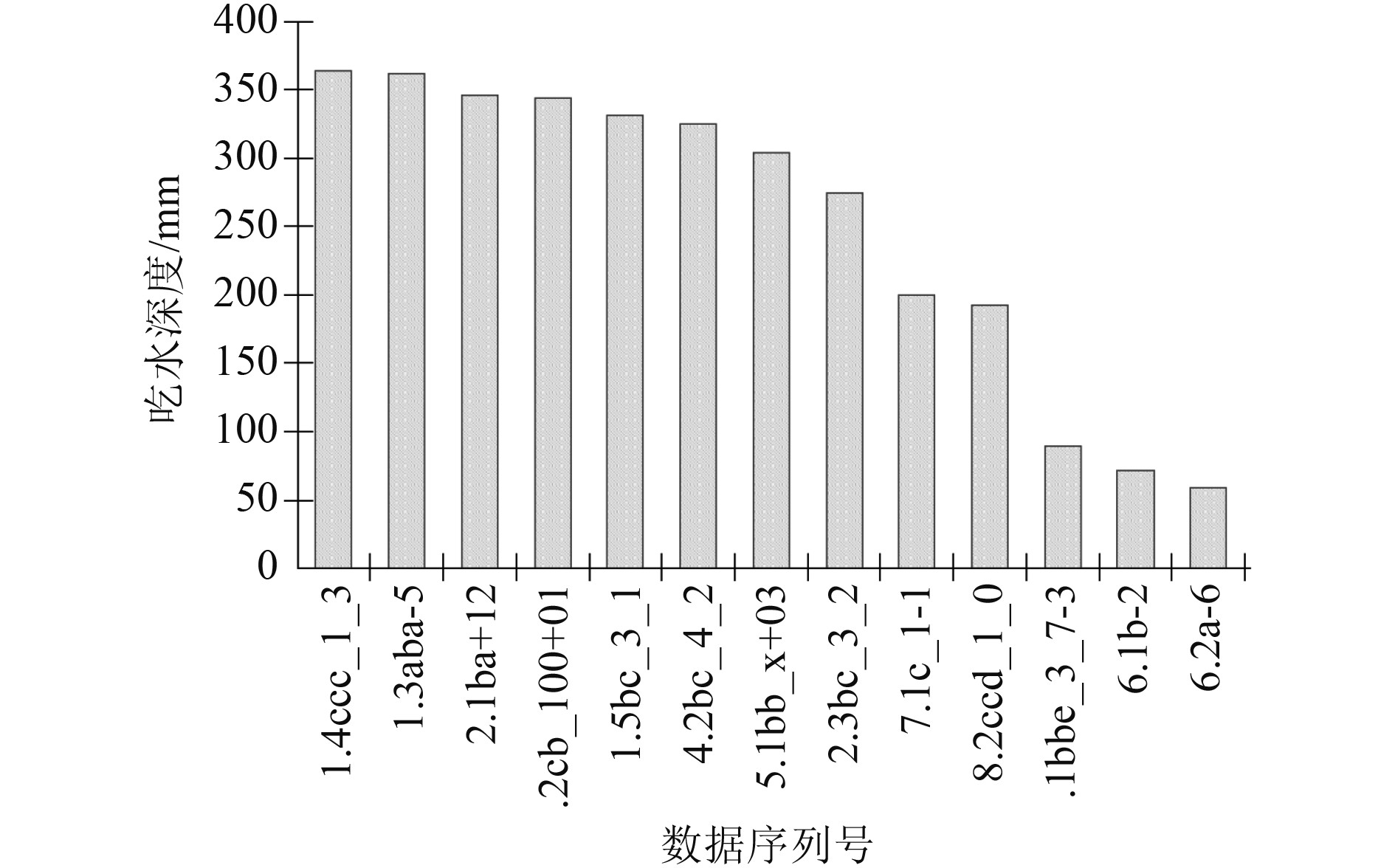图 5 吃水深度离散数据的统计直方图 Fig. 5 Statistical histogram of discrete data of draft depth

3）设定初始阈值

 ${g_0} = \frac{{{g_{\max }} + {h_{\min }}}}{2} \text{。}$

4）按照点云数据与阈值的对比将数据划分为高于阈值的数据和低于阈值的数据，并分别求2组数据的期望值。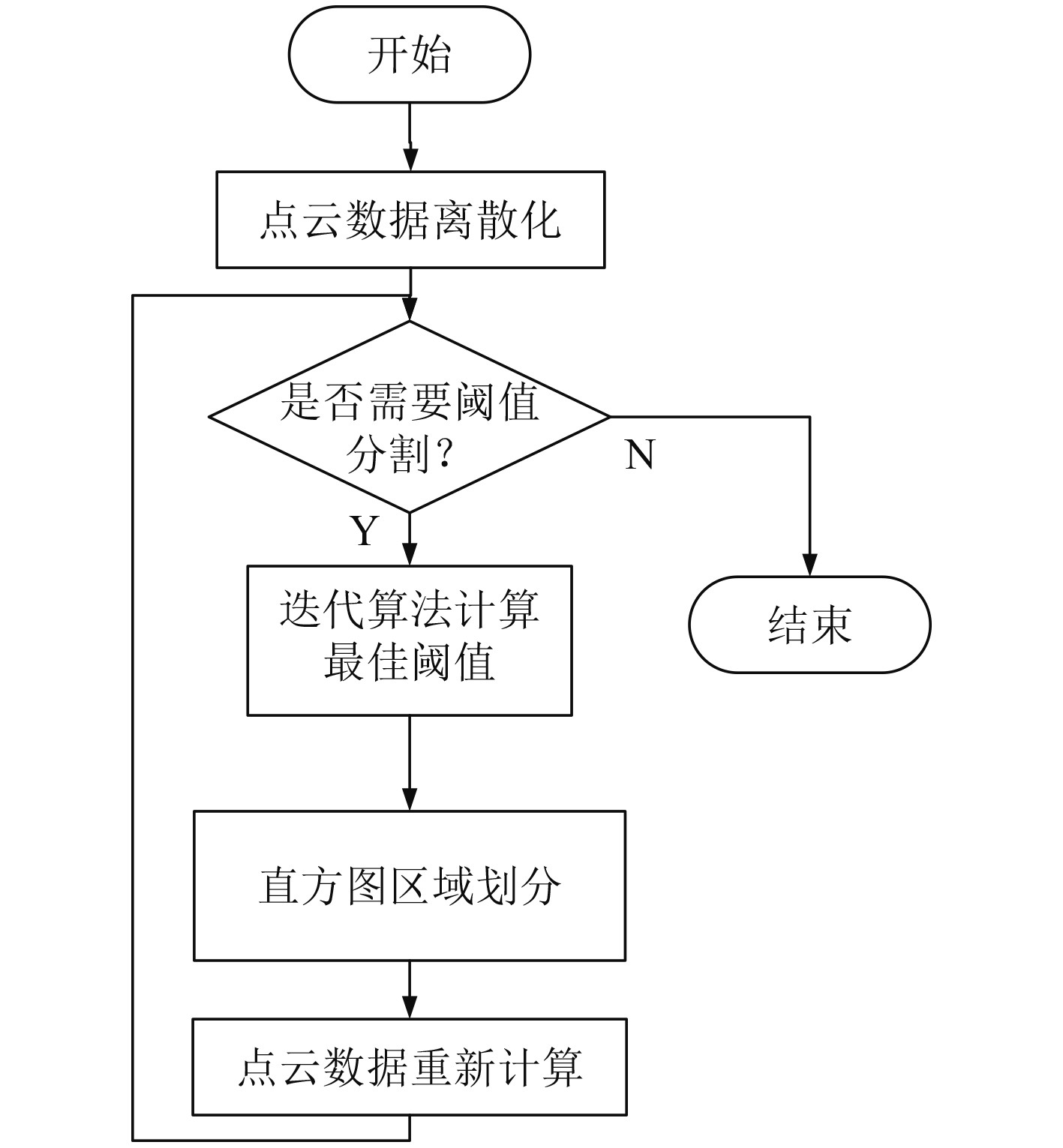图 6 基于最佳阈值的船舶吃水数据挖掘流程 Fig. 6 Ship draft data mining process based on optimal threshold
2.2 船体吃水数据的最小二乘法直线回归预测

 $\hat g(x) = ax + b \text{，}$

 $Q = \sum {{{\left( {{g_i} - a{x_i} - b} \right)}^2}} \text{，}$

 $\begin{gathered} \left( {\sum {x_i^2} } \right)a + \left( {\sum {{x_i}} } \right)b - \sum {{g_i}} {x_i} = 0 ，\hfill \\ \left( {\sum {{x_i}} } \right)a + nb - \sum {{g_i}} = 0 。\hfill \\ \end{gathered}$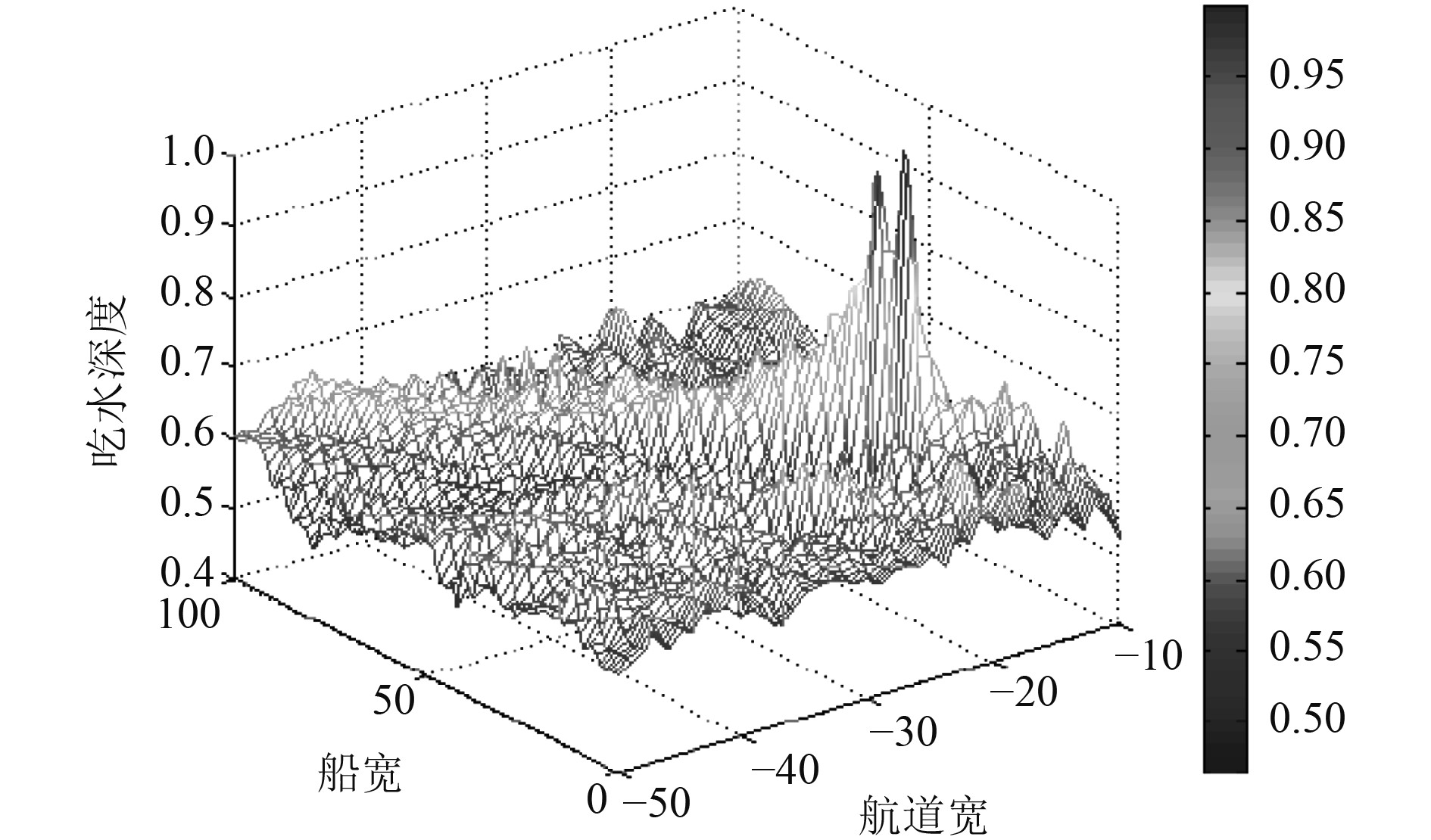图 7 监测系统在一段时间内的船体吃水深度预测3D图 Fig. 7 3D chart of hull draft prediction of monitoring system in a period of time
3 结　语

  陈宁, 秦建敏, 李国宏, 等. "最小二乘法变点冰水情数据处理算法"的研究与应用[J]. 数学的实践与认识, 2012, 42(1): 108-114.  刘志青, 李鹏程, 郭海涛, 等. 融合强阈值三角网与总体最小二乘曲面拟合滤波[J]. 红外与激光工程, 2016, 45(4): 135-142.  车金锐, 梁刚, 陈敏茹. 基于小波测量预处理的最小二乘估计[J]. 数学的实践与认识, 2006(4): 98-101.  张剑清. 基于特征的最小二乘匹配理论精度[J]. 武汉测绘科技大学学报, 1988(4): 82-90.